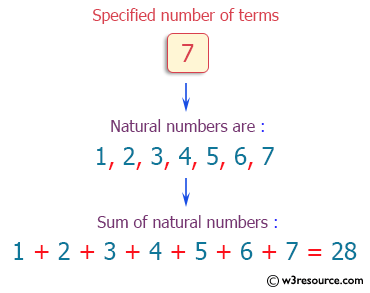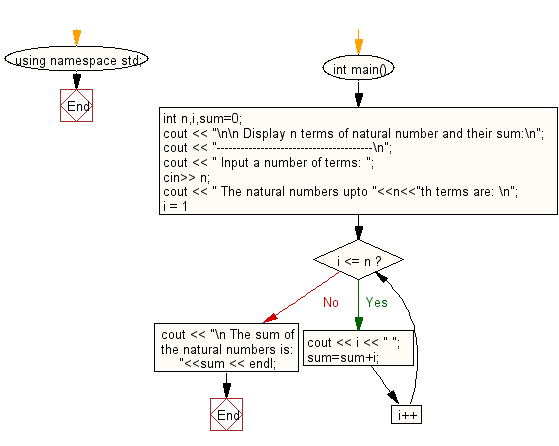﻿ C++ Exercises: Display n terms of natural number and their sum - w3resource# C++ Exercises: Display n terms of natural number and their sum

## C++ For Loop: Exercise-3 with Solution

Write a program in C++ to display n terms of natural number and their sum.

Pictorial Presentation:Sample Solution :-

C++ Code :

``````#include <iostream>
using namespace std;
int main()
{
int n,i,sum=0;
cout << "\n\n Display n terms of natural number and their sum:\n";
cout << "---------------------------------------\n";
cout << " Input a number of terms: ";
cin>> n;
cout << " The natural numbers upto "<<n<<"th terms are: \n";
for (i = 1; i <= n; i++)
{
cout << i << " ";
sum=sum+i;
}
cout << "\n The sum of the natural numbers is: "<<sum << endl;
}
``````

Sample Output:

``` Display n terms of natural number and their sum:
---------------------------------------
Input a number of terms: 7
The natural numbers upto 7th terms are:
1 2 3 4 5 6 7
The sum of the natural numbers is: 28
```

Flowchart:C++ Code Editor:

Contribute your code and comments through Disqus.

What is the difficulty level of this exercise?

﻿